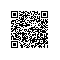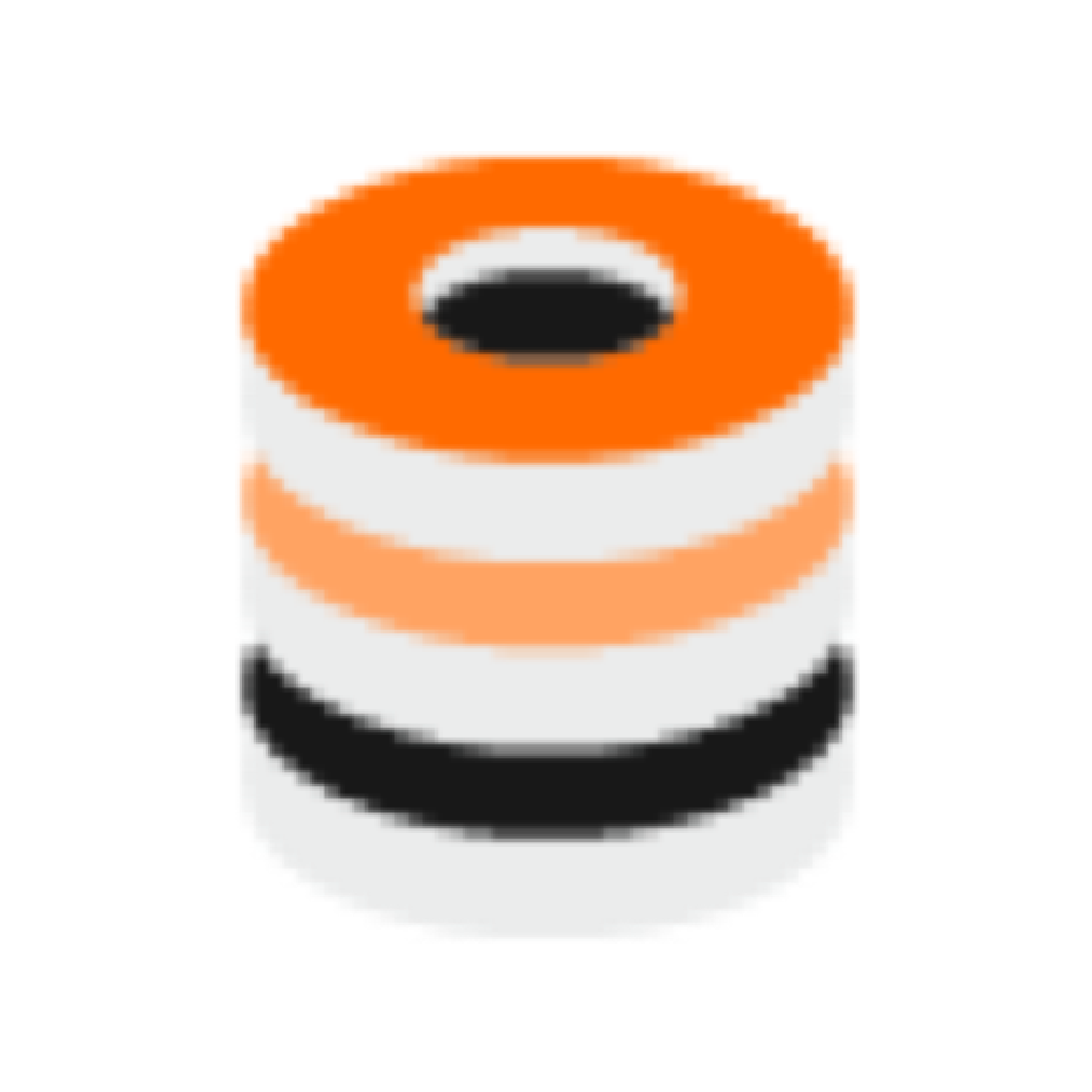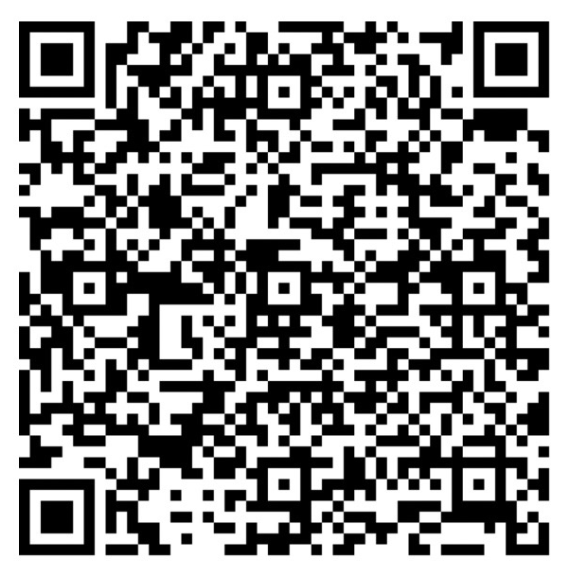# FineReport层式报表解决大数据集展示问题攻略ds1 里的内容如下$P=20$page=1;

ds2里的内容如下var toolbar = contentPane.toolbar;

var items = toolbar.options.items;

var customButton=items;//JQUERY取我们的工具栏上的按钮.items代表的是第三个，也就是那个显示成文本框按钮。

var inner = customButton.$table; var btnWrapper =$("em", inner);

btnWrapper.html("<input data-role='none' type='text'  class='fr-texteditor' style='width: 42px; height: 16px; text-align: center;'>");

//修正这个按钮的属性，让它变成文本类型，居中，鼠标放上次图标是编辑状态。

var cellValue = contentPane.curLGP.getCellValue("D1");//获取D1单元格的值就是page,也就是当前页。

var $input =$("input", btnWrapper);

$input.val(cellValue);//给这个文本控件复制，用的是JQUERY的方法，可以COPY。 var total=contentPane.curLGP.getCellValue("C1"); if (total>parseInt(total)){total=parseInt(total)+1; } contentPane.toolbar.options.items.setText(total); //把总页数显示在第5个控件上，因为这个数值可能是小数，所以判断下要不要加一处理。 contentPane.toolbar.options.items.setText(contentPane.curLGP.getCellValue("E1")); //把斜线写在第4个控件上。 7个自定义按钮： 第一个首页： 代码如下： window.location.href="${servletURL}?reportlet=fenye.cpt&op=write&page=1";//链接到fenye.cpt，page 参数=1，代表第一页。

var page= $("tr[tridx=0]","div.content-container").children().eq(0).html(); //取第一行第一个单元格内容。 if(page==0) { this.setEnable(false); alert("页面超出指定的范围"); } else window.location.href="${servletURL}?reportlet=fenye.cpt&op=write&page="+page//如果没有小于1就正常跳转，否则显示页面超过指定的范围，这个控件设置不可以使用。

var toolbar = contentPane.toolbar;

var items = toolbar.options.items;

var customButton=items;

var inner = customButton.$table; var btnWrapper =$("em", inner);

var $input =$("input", btnWrapper);

//取这个控件

$input.blur(function(){ var toolbar = contentPane.toolbar; var items = toolbar.options.items; var customButton=items; var inner = customButton.$table;

var btnWrapper = $("em", inner); var$input = $("input", btnWrapper); var page=$input.val();

var total=$("tr[tridx=0]","div.content-container").children().eq(2).html(); if (total>parseInt(total)){total=parseInt(total)+1; } if(parseInt(page) > parseInt(total) || parseInt(page) < parseInt(1) ) { alert("你输出的页数不再指定范围内"); } else window.location.href="${servletURL}?reportlet=fenye.cpt&op=write&page="+page

});

//失去焦点后输入的值是不是在指定的范围内，如果在跳转到指定页面，就在else后面的URL，否则输出你的输出页面不对提示。失去焦点代表就是点击其他地方或者点击TAB键。

var page= $("tr[tridx=0]","div.content-container").children().eq(1).html(); var total=$("tr[tridx=0]","div.content-container").children().eq(2).html();
//JQURUY取下一页和总页数
if (total>parseInt(total)){total=parseInt(total)+1;
}
//判断总页数是不是整数，不是加一
if(parseInt(page) > parseInt(total))
{
this.setEnable(false);
}
else
window.location.href="${servletURL}?reportlet=fenye.cpt&op=write&page="+page //如果下一页在这个范围就跳转到那里 这个控件不可用，输出出错。 第七个末页： 代码如下: var total=$("tr[tridx=0]","div.content-container").children().eq(2).html();
//取总页数。
if (total>parseInt(total)){total=parseInt(total)+1;
}
window.location.href="\${servletURL}?reportlet=fenye.cpt&op=write&page="+total
//判断总页数是不是整数，不是加一，并跳转到最后一页。使用钉钉扫一扫加入圈子
+ 订阅